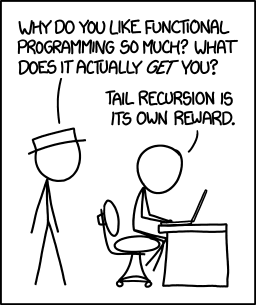Picking Out Elements Using a Logical List
08-12-2015, 03:05 AM
Post: #1Eddie W. ShoreSenior Member Posts: 979 Joined: Dec 2013
Picking Out Elements Using a Logical List
I am taking an online class in R Programming language (http://www.edx.org), having a great time. The program BOOLLIST is based on the ability in R to pick out elements using logical elements (TRUE, FALSE).

Example (in R):

vector <- [2, 3, 4, 5]
vector[ c(TRUE, TRUE, FALSE, TRUE) ] returns [2, 3, 5]

The logical vector doesn’t have to be same length as the source vector. If the logical vector has elements than the source vector.

vector <- [1, 2, 3, 4, 5, 6]
vector[ c(TRUE, FALSE, TRUE, FALSE, TRUE, FALSE) ] returns [1, 3, 5]
vector[ c(TRUE, FALSE) ] returns [1, 3, 5] (TRUE, FALSE pattern recycles)

Program BOOLLIST:

Input: BOOLLIST(source list, logical list)

Notes: Use list brackets { }. For the logical list, use 1 for TRUE and 0 for FALSE.

Code:
EXPORT BOOLLIST(LA, LB)
BEGIN

LOCAL LC, n, s, k, j;

//  Initialization
LC≔{ };
j≔1;
s≔SIZE(LA);
b≔SIZE(LB);

// Process
FOR k FROM 1 TO s DO

IF LB(j)==1 THEN
LC≔CONCAT(LC,LA(k));
END;

j≔j+1;

IF j>n THEN
j≔1;
END;

END;

RETURN LC;

END;

Examples:

In addition to the examples above that can be tried with BOOLLIST:

BOOLLIST( {4,2,3,6}, {1,0,0,1} ) returns {4, 6}

BOOLLIST( {3,9,6,-1,6}, {1,0} ) returns {3, 6, 6}

http://edspi31415.blogspot.com/2015/08/h...using.html
08-12-2015, 10:40 AM
Post: #2
 Didier LachiezeSenior Member Posts: 1,143 Joined: Dec 2013
RE: Picking Out Elements Using a Logical List
Nice function ! I was expecting it would be very easy to implement it with the current Prime list functions but I've not found a simple solution without a FOR loop.
So, here is another way to do it:

Code:
EXPORT BOOLLIST(LA,LB)
BEGIN
LOCAL LC,k;
LC:={};
FOR k FROM 0 TO SIZE(LA)-1 DO
IF LB(k MOD SIZE(LB)+1) THEN
LC(0):=LA(k+1);
END;
END;
RETURN LC;
END;

Note: storing to LC(0) is used to add an element to the list LC.
08-16-2015, 11:53 AM
Post: #3
 roadrunnerMember Posts: 276 Joined: Jun 2015
RE: Picking Out Elements Using a Logical List
Here is a method without a for loop:

Code:

export BOOLLIST3(LA,LB)
BEGIN
local LC:={};
local s:=size(LA);
IF LB(1) THEN LC(0):=LA(1);END;
IF s==1 THEN return LC;end;
LC:=concat(LC,BOOLLIST3(SUB(LA,2,s),CONCAT(SUB(LB,2,SIZE(LB)),LB(1))));
END;

Don't know if it qualifies as simple or not; it runs substantially slower than your version.
08-16-2015, 11:40 PM
Post: #4
 roadrunnerMember Posts: 276 Joined: Jun 2015
RE: Picking Out Elements Using a Logical List
And another version using no declared variables and no for loop:

Code:
export BOOLLIST4(LA,LB)
BEGIN
IF LB(1) THEN
IF size(LA)==1 THEN return LA(1);end;
return concat(LA(1),BOOLLIST4(SUB(LA,2,size(LA)),CONCAT(SUB(LB,2,SIZE(LB)),LB(1)))​);
end;
if size(LA)==1 then return {};end;
BOOLLIST4(SUB(LA,2,size(LA)),CONCAT(SUB(LB,2,SIZE(LB)),LB(1)));
END;
08-17-2015, 10:56 AM (This post was last modified: 08-17-2015 11:01 AM by Didier Lachieze.)
Post: #5
 Didier LachiezeSenior Member Posts: 1,143 Joined: Dec 2013
RE: Picking Out Elements Using a Logical List
Nice idea to use the recursion to solve this problem. Here is a variation of your first program:
Code:
EXPORT BOOLLIST5(LA,LB)
BEGIN
LOCAL LC:={};
IF LB(1) THEN LC(1):=LA(1); END;
IF SIZE(LA)==1 THEN RETURN LC; END;
LB(0):=LB(1);
CONCAT(LC,BOOLLIST5(tail(LA),tail(LB)));
END;
08-17-2015, 11:32 AM
Post: #6
 Thomas KlemmSenior Member Posts: 1,448 Joined: Dec 2013
RE: Picking Out Elements Using a Logical List
(08-17-2015 10:56 AM)Didier Lachieze Wrote:  Nice idea to use the recursion to solve this problem.08-18-2015, 12:13 PM
Post: #7
 roadrunnerMember Posts: 276 Joined: Jun 2015
RE: Picking Out Elements Using a Logical List
(08-17-2015 10:56 AM)Didier Lachieze Wrote:  Here is a variation of your first program
I was not aware of the tail function (nor the head function). Thank you for sharing.
 « Next Oldest | Next Newest »

User(s) browsing this thread: 1 Guest(s)